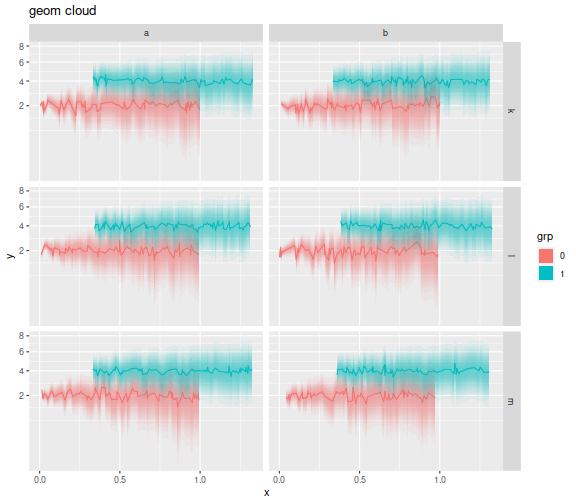I wanted a drop-in replacement for `geom_errorbar` in `ggplot2` that would plot a density cloud of uncertainty. The idea is that typically (well, where I work), the `ymin` and `ymax` of an errorbar are plotted at plus and minus one standard deviation. A 'cloud' where the alpha is proportional to a normal density with the same standard deviations could show the same information on a plot with a little less clutter. I found out how to do this with a very ugly function, but wanted to do it the 'right' way by spawning my own geom. So the `geom_cloud`.

After looking at a bunch of other `ggplot2` extensions, some amount of tinkering and hair-pulling, and we have the following code. The first part just computes standard deviations which are equally spaced in normal density. This is then used to create a list of `geom_ribbon` with equal alpha, but the right size. A little trickery is used to get the scales right. There are three parameters: the `steps`, which control how many ribbons are drawn. The default value is a little conservative. A larger value, like 15, gives very smooth clouds. The `se_mult` is the number of standard deviations that the `ymax` and `ymin` are plotted at, defaulting to 1 here. If you plot your errorbars at 2 standard errors, change this to 2. The `max_alpha` is the alpha at the maximal density, i.e. around `y`.

```# get points equally spaced in density
equal_ses <- function(steps) {
xend <- c(0,4)
endpnts <- dnorm(xend)
deql <- seq(from=endpnts,to=endpnts,length.out=steps+1)
davg <- (deql[-1] + deql[-length(deql)])/2
# invert
xeql <- unlist(lapply(davg,function(d) {
uniroot(f=function(x) { dnorm(x) - d },interval=xend)\$root
}))
xeql
}

library(ggplot2)
library(grid)

geom_cloud <- function(mapping = NULL, data = NULL, ...,
na.rm = TRUE,
steps = 7, se_mult=1, max_alpha=1,
inherit.aes = TRUE) {

layer(
data = data,
mapping = mapping,
stat = "identity",
geom = GeomCloud,
position = "identity",
inherit.aes = inherit.aes,
params = list(
na.rm = na.rm,
steps = steps,
se_mult = se_mult,
max_alpha = max_alpha,
...
)
)
}

GeomCloud <- ggproto("GeomCloud", Geom,
required_aes = c("x", "y", "ymin", "ymax"),
non_missing_aes = c("fill"),
default_aes = aes(
colour = NA, fill = NA, alpha = 1, size=1, linetype=1
),
setup_data = function(data,params) {
if (params\$na.rm) {
ok_row <- !(is.na(data\$x) | is.na(data\$y) | (is.na(data\$ymin) & is.na(data\$ymax)))
data <- data[ok_row,]
}
ses <- equal_ses(params\$steps)
data\$up_se <- (1/params\$se_mult) * (data\$ymax - data\$y)
data\$dn_se <- (1/params\$se_mult) * (data\$y - data\$ymin)
# a trick to get positions ok: puff up the ymax and ymin for now
maxse <- max(ses)
data\$ymax  <- data\$y + maxse * data\$up_se
data\$ymin  <- data\$y - maxse * data\$dn_se
data
},
draw_group = function(data, panel_scales, coord,
na.rm = TRUE,
steps = 5, se_mult=1, max_alpha=1) {

data\$alpha <- max_alpha / steps
# 2FIX: use the coordinate transform? or just forget it?
ses <- equal_ses(steps)
grobs <- Map(function(myse) {
this_data <- data
this_data\$ymax <- this_data\$y + myse * this_data\$up_se
this_data\$ymin <- this_data\$y - myse * this_data\$dn_se
ggplot2::GeomRibbon\$draw_group(this_data, panel_scales, coord, na.rm=na.rm)
},ses)
do.call("gList",grobs)
},
draw_key = draw_key_polygon
)
```

Ok, now I use it. I construct some data in multiple groups, splitting into column and row facets by some random variable. Within each facet there are two groups. The standard error is proportional to square root x. I then plot the clouds. I put these in square root space to convince myself I had not goofed up scale transformations. (Well, still not sure, really.)

```library(dplyr)

nobs <- 1000

set.seed(2134)
mydat <- data.frame(grp=sample(c(0,1),nobs,replace=TRUE),
colfac=sample(letters[1:2],nobs,replace=TRUE),
rowfac=sample(letters[10 + (1:3)],nobs,replace=TRUE)) %>%
mutate(x=seq(0,1,length.out=nobs) + 0.33 * grp) %>%
mutate(y=0.25*rnorm(nobs) + 2*grp) %>%
mutate(grp=factor(grp)) %>%
mutate(se=sqrt(x)) %>%
mutate(ymin=y-se,ymax=y+se)

offs <- 2
ph <- mydat %>%
mutate(y=y+offs,ymin=ymin+offs,ymax=ymax+offs) %>%
ggplot(aes(x=x,y=y,ymin=ymin,ymax=ymax,color=grp,fill=grp)) +
facet_grid(rowfac ~ colfac) +
scale_y_sqrt() + geom_line() +
geom_cloud(aes(fill=grp),steps=15,max_alpha=0.85,color=NA) +   # important!  set color to NA!
labs(title='geom cloud')
print(ph)
```At some point I will put this in a package or try to push it off into one of the fine `ggplot2` extension packages. There are still some bugs to be worked out: I would like the fill to default to the color if the fill is not given. I want to force the color to be `NA`, otherwise `geom_ribbon` adds really ugly lines, and so on.